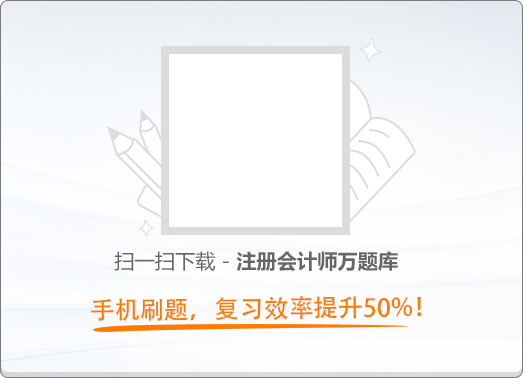150:002016年注册会计师《公司战略与风险管理》真题

1
(单项选择题)

• A.

多元化战略

• B.

差异化战略

• C.

一体化战略

• D.

产品开发战略

• A
• B
• C
• D

2
(单项选择题)

• A.

跨越时间

• B.

跨越战略群体

• C.

重新界定产业的买方群体

• D.

重设客户的功能性或情感性诉求

• A
• B
• C
• D

3
(单项选择题)

• A.

库存生产式生产

• B.

订单生产式生产

• C.

资源订单式生产

• D.

准时生产式生产

• A
• B
• C
• D

4
(单项选择题)

• A.

跨国战略

• B.

国际战略

• C.

全球化战略

• D.

多国本土化战略

• A
• B
• C
• D

5
(单项选择题)

• A.

防御者

• B.

扩张者

• C.

躲闪者

• D.

抗衡者

• A
• B
• C
• D

6
(单项选择题)

• A.

物理上独特的资源

• B.

具有经济制约性的资源

• C.

具有因果含糊性的资源

• D.

具有路径依赖性的资源

• A
• B
• C
• D

7
(单项选择题)

M国的甲航空公司专营国内城际航线，以低成本战略取得很大成功。专营H国国内城际航线的H国乙航空公司，也采用低成本战略，学习甲公司的成本控制措施，在H国竞争激烈的航空市场取得了良好的业绩。乙公司基准分析的类型是（　）。

• A.

内部基准

• B.

一般基准

• C.

竞争性基准

• D.

过程或活动基准

• A
• B
• C
• D

8
(单项选择题)

• A.

明星业务

• B.

问题业务

• C.

瘦狗业务

• D.

现金牛业务

• A
• B
• C
• D

9
(单项选择题)

• A.

增长型战略

• B.

多元化战略

• C.

扭转型战略

• D.

防御型战略

• A
• B
• C
• D

10
(单项选择题)

• A.

加强协同作用

• B.

重新制定战略

• C.

以企业使命为基础

• D.

根据文化进行管理

• A
• B
• C
• D

11
(单项选择题)

2002年，小王在市区黄金位置开了一家咖啡店。由于经营有方，小店开业不到一个月就创造了销售佳绩。正在小王准备大干一场时，社会上一场流行性疾病袭来，小店经营陷入困境。小王采取各种措施试图挽救失败后，不得不关闭了咖啡店。根据战略失效理论，小王创业没达到预期目标属于（　）。

• A.

前期失效

• B.

晚期失效

• C.

正常失效

• D.

偶然失效

• A
• B
• C
• D

12
(单项选择题)

• A.

重大投资项目应当按照规定的权限和程序实行集体决策或联签制度

• B.

转让投资必须委托具有相应资质的专门机构进行评估，并报授权批准部门批准

• C.

企业选择投资项目应当突出主业，不得从事股票投资或衍生金融产品等高风险投资

• D.

投资方案发生重大变更的，应视情况决定是否重新进行可行性研究并履行相应审批程序

• A
• B
• C
• D

13
(单项选择题)

• A.

经理层对董事会负责

• B.

经理层依法行使企业的经营决策权

• C.

监事会对股东（大）会负责，监督企业董事、经理和其他髙管依法履行职责

• D.

企业重大决策、重大事项，应当按照规定的权限和程序实行集体决策审批或联签制度

• A
• B
• C
• D

14
(单项选择题)

• A.

重大担保业务由总经理审批

• B.

企业的内设机构不得办理担保业务

• C.

在采取相应措施的情况下，可以给经营风险较大的企业进行担保

• D.

被担保人要求变更担保事项的，企业应当重新履行评估与审批程序

• A
• B
• C
• D

15
(单项选择题)

• A.

企业对外发生经济行为，必须订立书面合同

• B.

合同文本一般由法律部门起草，总经理审核

• C.

正式对外订立的合同，必须由企业法定代表人签名并加盖有关印章

• D.

企业对影响重大或法律关系复杂的合同文本，应该组织内部有关部门进行审核

• A
• B
• C
• D

16
(单项选择题)

• A.

实地查验是一个适合于企业层面评价的方法

• B.

审阅与检查是一个适合于业务层面评价的方法

• C.

个别访谈是一个适合于企业层面和业务层面评价的方法

• D.

抽样是从样本库抽取一定数量的样本，进行控制测试，以获取有关控制的运行情况

• A
• B
• C
• D

17
(单项选择题)

• A.

采购管理

• B.

技术开发

• C.

人力资源管理

• D.

公司基础设施

• A
• B
• C
• D

18
(单项选择题)

• A.

供应商提供的产品专用性程度越高，其讨价还价能力越强

• B.

供应商拥有足够的资源能够进行后向一体化时，其讨价还价能力强

• C.

供应商借助互联网平台掌握的购买者转换成本信息越多，其讨价还价能力越强

• D.

占市场份额80%以上的少数供应商将产品销售给较为零散的购买者时，其讨价还价能力强

• A
• B
• C
• D

19
(单项选择题)

• A.

市场风险

• B.

品牌风险

• C.

环境风险

• D.

文化风险

• A
• B
• C
• D

20
(单项选择题)

• A.

企业管理层及全体员工都未辨识出风险

• B.

企业面临影响企业目标实现的重大风险

• C.

企业从成本效益考虑认为选择风险承担是最适宜的方案

• D.

企业缺乏能力对已经辨识出的风险进行有效管理与控制

• A
• B
• C
• D

21
(单项选择题)

• A.

德尔菲法

• B.

头脑风暴法

• C.

情景分析法

• D.

因素分析法

• A
• B
• C
• D

22
(单项选择题)

• A.

ERP系统

• B.

SCM系统

• C.

CRM系统

• D.

MIS系统

• A
• B
• C
• D

23
(单项选择题)

• A.

共同价值观

• B.

标准化体系结构

• C.

直接指挥，直接控制

• D.

相互适应，自行调整

• A
• B
• C
• D

24
(单项选择题)

• A.

可利用三家粮油公司之间的竞争压低采购价格

• B.

有利于从三家粮油公司获得更多的知识和技术信息

• C.

有利于促使三家粮油公司提供有统一质量保证的产品

• D.

不会因某家粮油公司的供货问题而严重影响企业经营

• A
• B
• C
• D